﻿ First-Order Digital Filters for Second-Order Digital PLLs

# First-Order Digital Filters for Second-Order Digital PLLs

by Chip Fleming of Spectrum Applications

This is a short paper discussing two implementations of digital loop filters for phase-locked loops (PLLs) implemented either in digital circuitry or in software. This paper is by no means intended to be a comprehensive theoretical discussion of the topic, but rather a quick reference for the engineering student or practicing engineer. If you need a theoretical background in the subject of PLLs, please see the bibliography at the end of this monograph.

First form

Here is a block diagram of a first-order loop filter that I first saw in an article by Louis Litwin in RF Design Magazine (see his Figure 8):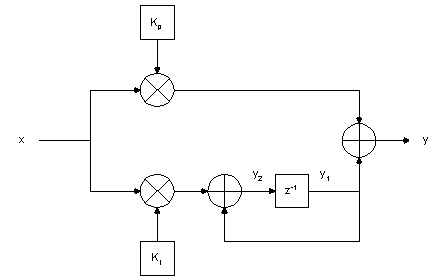Mr. Litwin calls this a "second-order loop filter," but it is actually a first-order loop filter for a second-order loop. The order is determined by the highest (negative) power of z in the denominator of the transfer function y/x, which in this case is one, as we will see shortly. The loop itself is second-order because the numerically-controlled oscillator forms a second integrator.

Let us examine this block diagram. The phase detector output, x, is multiplied by the proportional gain constant Kp in the upper arm. In the lower arm, the phase detector output is first multiplied by Ki , the integral gain constant. The result of this multiplication is fed into an integrator comprising an adder and a register (unit delay). The final output y is the sum of the product of the proportional gain constant K p and the phase error computed in the upper arm, and the output of the integrator in the lower arm.

Here is a derivation of the transfer function y/x of this loop filter. I introduced a couple of intermediate variables, y1 and y2, to make the job easier: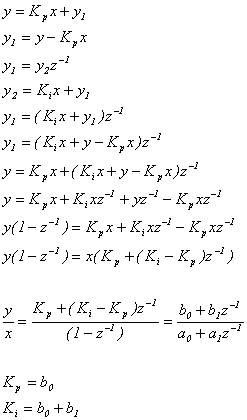This loop filter could be implemented in software using the following equation:  y(n) = Kp * x(n) + Ki * x(n-1) + y(n-1) - Kp * x(n-1), where y(n) is the current filter output, y(n-1) is the previous filter output, x(n) is the current phase detector output, and x(n-1) is the previous phase detector output.  The phase detector output is computed and the filter output updated every Ts seconds, where Ts  is the sampling interval.

And now let's see how we can compute b0 and b1 , starting with an analog prototype and then using the bilinear z transform: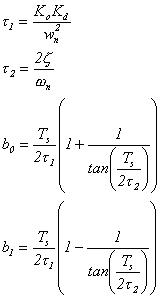In the equations above, Ko is the oscillator gain in radians sec-1 volt-1 in the analog domain, or radians sec-1 unit-1 in the digital domain.  Kd is the phase detector gain in volts radians-1 in the analog domain, or unit radians-1 in the digital domain.  The natural frequency of the loop is wn, and the damping factor is z.  Ts is the sampling interval of the digital system, and t1 and t2 are the loop time constants.  These equations are the same for all three types of first-order loop filters I describe herein, so I will not repeat them.

Second form

Here is a block diagram of another first-order loop filter that I have seen used in a couple of different places: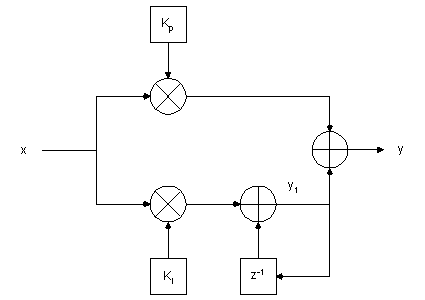This loop filter is very similar to the first loop filter, except that the output of the integrator in the lower arm is taken from the adder instead of from the register.  This makes a difference in the transfer function, which we will now derive: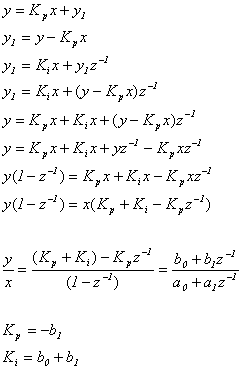You would implement this loop filter in software using the following equation:  y(n) = Kp * x(n) + Ki * x(n) + y(n-1) - Kp * x(n-1).

Bibliography

Gardner, Floyd M., Phaselock Techniques, New York:  John Wiley and Sons, Inc., 1966

Wolaver, Dan H., Phase-Locked Loop Circuit Design, New York:  Prentice Hall, 1991

Best, Roland E., Phase-Locked Loops:  Design, Simulation, and Applications, New York:  McGraw-Hill Professional Publishing, 1999 (but wait for my comments to be addressed in the 5th edition before buying this one ;-)